Author: Oscar Cronquist Article last updated on March 17, 2023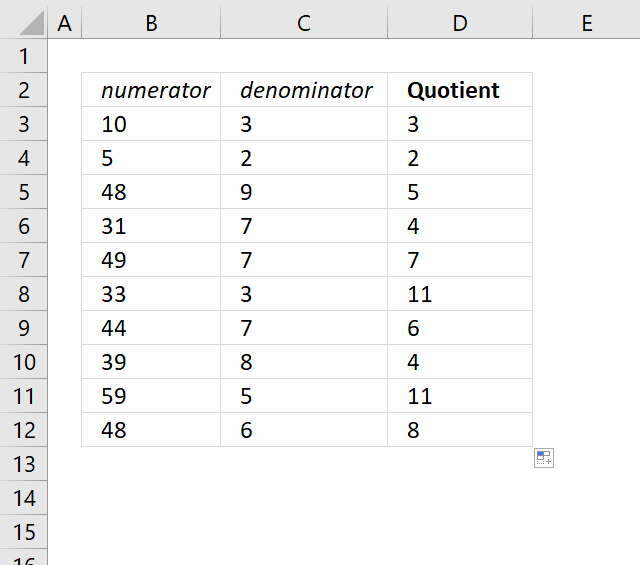The quotient function returns the integer portion of a division.

Example, 5/2 = 2.5. The integer is 2.

## 1. QUOTIENT Function Syntax

QUOTIENT(numerator, denominator)

## 2. QUOTIENT Function Arguments

 numerator Required. The dividend. denominator Required. The divisor.

The QUOTIENT function returns the #VALUE! if the arguments are not numbers.

The following formula is equivalent to the QUOTIENT function:

=INT(numerator/denominator)

## 3. How to remove decimals from a division?You are about to send your company products to customers. You have boxes you can send the products in.

You have 18 items of product A in your inventory (cell B2). The box for product A can hold up to 4 items (cell C2).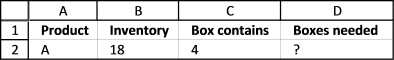How many boxes do you need for product A?

Yes, you can build a formula for that but you don't need to do that, there is a function in Excel. Let me introduce the Quotient function.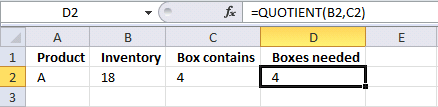The quotient function returns the integer portion of a division. Simple as that.

QUOTIENT(numerator, denominator)

There are two arguments, the numerator is the dividend and the denominator is the divisor.

The formula in cell D2:

=QUOTIENT(B2,C2)

returns 4.

If you divide 18 by 4, you get 4.5.

The Quotient function returns 4 because the integer part of 4.5 is 4.

## 4. How to remove decimals from a number?

There is an Integer function also in excel so this formula gives the same result:

=INT(B2/C2)

The INT function rounds a number down to the nearest integer.

## 5. How to calculate the remainder?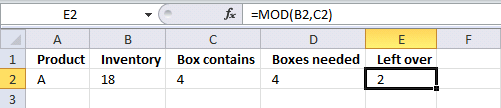The next question is how many products are left? Believe it or not, there is a function for that too.

The Mod function returns the remainder after a number is divided by divisor.

Formula in cell E2:

=MOD(B2, C2)

18 divided by 4 is 4.5. The integer part of 4.5 is 4. 4 x 4 is 16. 18-16 is 2.
2 products are left.

You can use the mod function in a conditional formatting formula to highlight every n-th row:

Recommended articles

How to use the MOD function
The Mod function returns the remainder after a number is divided by a divisor. The Mod function is short for […]

## 6. Is there a division function in Excel?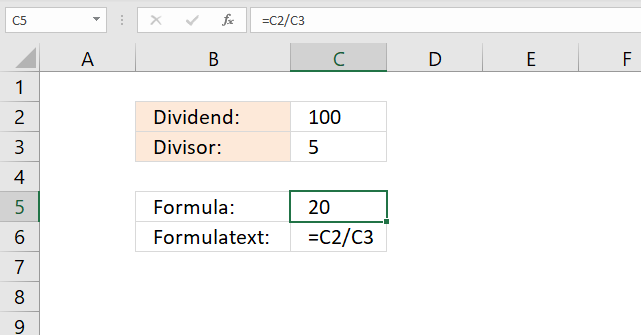There is no division function in Excel, however, you can use the slash character to calculate a division. The image above demonstrates a formula in cell C5 that divides the number in cell C2 with the number in cell C3.

Formula in cell C5:

=C2/C3

C2 and C3 are cell references.

C2/C3

becomes

100/5

and returns 20 in cell C5.

## 7. How do you divide and round up in Excel?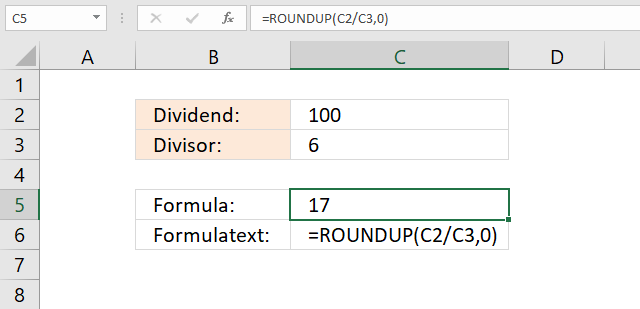The image above shows a formula in cell C5 that divides two numbers and then rounds up the result.

Formula in cell C5:

=ROUNDUP(C2/C3,0)

### Explaining formula in cell C5

#### Step 1 - Division

The slash character lets you divide two numbers in an Excel formula.

C2/C3

becomes

100/6

and returns approx 16.6666666666667.

#### Step 2 - Round the number up to the nearest integer

The ROUNDUP function calculates a number rounded up based on the number of digits to which you want to round the number.

ROUNDUP(numbernum_digits)

ROUNDUP(C2/C3,0)

becomes

ROUNDUP( 16.6666666666667,0)

and returns 17.

## 8. How to find divisors of a number that return no remainders?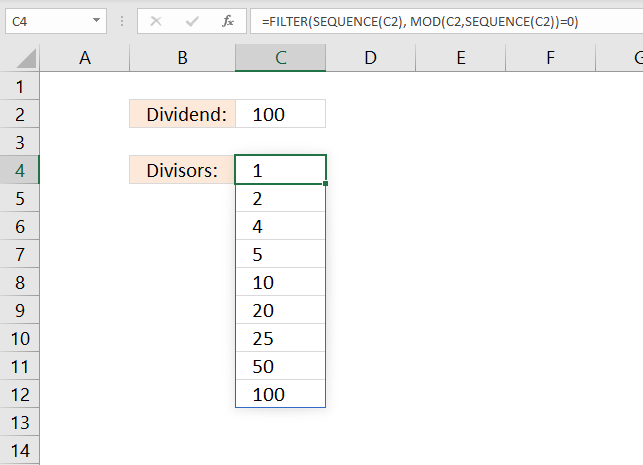The formula in cell C4 shown in the image above is a dynamic array formula that automatically spills values to cells below. It calculates all divisors that can be used so the result returns a whole number based on the dividend specified in cell C2.

Example, number 5 returns no remainders. 100/5 = 20. The result is a whole number. Change the number in cell C2 and Excel automatically calculates divisors in cell C4 and cells below. There is a dividend limit of 1048576, you can't go higher than that.

Excel 365 formula in cell C4:

=FILTER(SEQUENCE(C2), MOD(C2,SEQUENCE(C2))=0)

The following formula is an array formula, it works for all previous Excel versions and returns the same numbers as the Excel 365 formula.

Array formula for previous Excel versions:

=SMALL(IF(MOD(\$C\$2,SEQUENCE(\$C\$2))=0,SEQUENCE(\$C\$2),""),ROWS(\$A\$1:A1))

### How to enter an array formula

1. Double press with left mouse button on with left mouse button on cell C4.
2. Paste the formula.
3. Press and hold CTRL + SHIFT keys simultaneously.
4. Press Enter once.
5. Release all keys.

Copy cell C4 and paste to cells below as far as needed. This is required if you are using the array formula.

### Explaining Excel 365 formula in cell C4

#### Step 1 - Create a sequence

The SEQUENCE function returns an array containing a sequence of numbers.

SEQUENCE(C2)

becomes

SEQUENCE(100)

and returns {1; 2; 3; ... 98; 99; 100}. Not all numbers are shown in this sequence.

#### Step 2 - Calculate remainders

The MOD function returns the remainder of a division.

MOD(\$C\$2,SEQUENCE(\$C\$2))

becomes

MOD(100, {1; 2; 3; ... 98; 99; 100})

and returns {0 ;0 ;1 ; ... ; 2; 1; 0}.

#### Step 3 - Create a logical expression

The equal sign allows you to compare the numbers in the array to 0 (zero). The result is boolean values TRUE or FALSE.

MOD(C2,SEQUENCE(C2))=0

becomes

{0 ;0 ;1 ; ... ; 2; 1; 0}=0

and returns {TRUE ;TRUE ;FALSE; ... ; FALSE; FALSE; TRUE }.

#### Step 4 - Filter values based on logical expression

The FILTER function lets you extract values/rows based on a condition or criteria.

FILTER(SEQUENCE(C2), MOD(C2,SEQUENCE(C2))=0)

becomes

FILTER(SEQUENCE(C2), {TRUE ;TRUE ;FALSE; ... ; FALSE; FALSE; TRUE })

becomes

FILTER({1; 2; 3; ... 98; 99; 100}, {TRUE ;TRUE ;FALSE; ... ; FALSE; FALSE; TRUE })

and returns {1; 2; 4; 5; 10; 20; 25; 50; 100}.

## 9. How to create a repeating sequence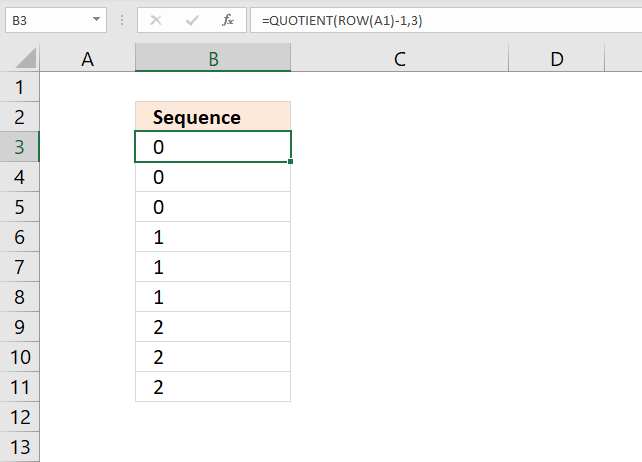The image above demonstrates a formula that returns repeating numbers in a sequence. The second argument determines how many times a number is repeated before moving on to the next number.

Formula in cell B3:

=QUOTIENT(ROWS(\$A\$1:A1)-1,3)

Change the formula to this if you want the sequence to start with 1 instead of 0 (zero).

=QUOTIENT(ROWS(\$A\$1:A4)-1,3)

### Explaining formula in cell B3

#### Step 1 - Calculate a number that changes based on a relative and absolute cell ref

The ROWS function returns a number representing the number of rows in cell reference. Cell ref \$A\$1:A1 is a growing cell reference that changes when you copy the cell and paste to cells below.

It contains an absolute (\$A\$1) indicated by the dollar signs and a relative part (A1).

ROWS(\$A\$1:A1)-1

becomes

1-1

amd returns 0 (zero).

#### Step 2 - Calculate quotient

QUOTIENT(numerator, denominator)

QUOTIENT(ROWS(\$A\$1:A1)-1,3)

becomes

QUOTIENT(0,3)

and returns 0 (zero) in cell B3.